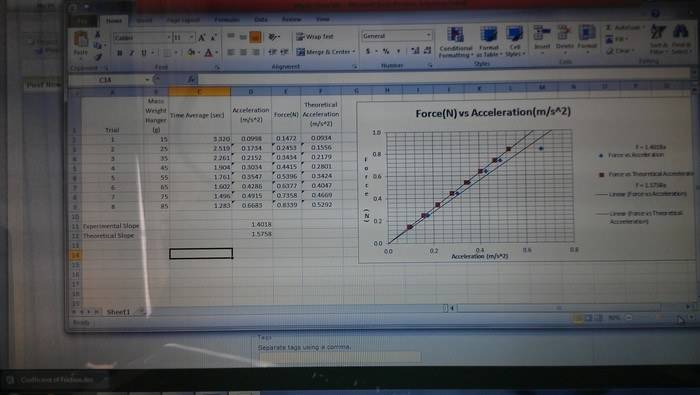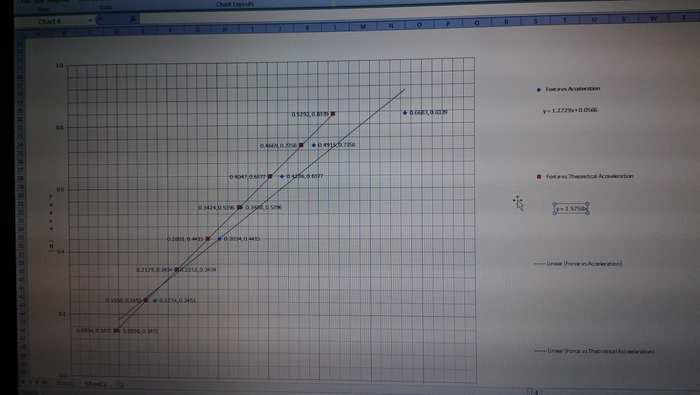# How to determine the force of friction from a graph?

Arooj

## Homework Statement

I had to a lab on the Newton's second law of motion, and one of the direction was to "determine the force of friction" from a graph I constructed. The experiment is similar to this: http://people.sc.fsu.edu/~dduke/manual/gp1.htm [Broken]
This image of the data is a lot cleaner than my representation of it.Data:
Trial Mass Weight Hanger (g) Time Average (sec) Acceleration (m/s^2) Force(N) Theoretical Acceleration (m/s^2)
1 15 3.320 0.0998 0.1472 0.0934
2 25 2.519 0.1734 0.2453 0.1556
3 35 2.261 0.2152 0.3434 0.2179
4 45 1.904 0.3034 0.4415 0.2801
5 55 1.761 0.3547 0.5396 0.3424
6 65 1.602 0.4286 0.6377 0.4047
7 75 1.496 0.4915 0.7358 0.4669
8 85 1.283 0.6683 0.8339 0.5292

Experimental Slope 1.4018
Theoretical Slope 1.5758
Total mass of system 1.5758 kg

## Homework Equations

F = uN = umg ( But we weren't given a coefficient of friction, so I wonder if calculating frictional force is even possible)
Fk = -F (Fk = kinetic force of friction)

## The Attempt at a Solution

I think you would subtract 1.5758 - 1.4018 to get the difference in kg, and then multiply that by an acceleration of 1 to get the coefficient of friction 0.174. Then I guess you multiply that by the 1.5758 to get the force of friction 0.2742 N.

Last edited by a moderator:

## Answers and Replies

Homework Helper
Gold Member
You want to get the force of friction from your graph rather than by calculation. So, you need to consider how friction affects the look of the graph. To do this, write out Newton's 2nd law (symbolically) for the horizontal motion of the cart including friction. Solve the equation for the applied force and interpret in terms of what the equation would look like if you graphed the applied force vs acceleration when friction is present.

Arooj
What values would I use for the mass and acceleration to find the applied force? The total mass of the system is 1.5758 kg. So then F = 1.5758a, which gets me back to the equation of the line for theoretical acceleration. Do you take the average of all the accelerations?

Last edited:
Homework Helper
Gold Member
Don't plug in any numbers. Just consider the equation (in symbols) that would express the applied force in terms of the mass, acceleration and the friction force. Then you can tell how the friction force can be obtained from the graph without any calculation. To get the equation, set up Newton's second law and solve for the applied force.

Arooj
F = ma?

Homework Helper
Gold Member
Yes, but the left hand side must represent the net force. How would you write the net force in terms of the applied force and the friction force?

Arooj
applied force - friction force = net force
net force = applied force - friction force
applied force - friction force = ma ?

Homework Helper
Gold Member
Now solve for the applied force. Your graph is a plot of the applied force on the "y" axis and acceleration on the "x" axis. Does your equation for the applied force have the form y = mx+b? If so, how does the force of friction affect the graph?

Arooj
y = mx + b
applied force = ma + friction force
It causes an increase in force?

Homework Helper
Gold Member
What does b represent in y = mx + b?

Arooj
friction force, wouldn't that value have to be negative though because it opposes the applied force?

Homework Helper
Gold Member
You took care of that when you wrote the net force as Fapplied minus Ffriction. Rearranging makes the friction force appear as a positive contribution to the right hand side.

Arooj
Ah, so the frictional force is just -0.174?

Homework Helper
Gold Member
Where did you get that number?

Homework Helper
Gold Member
Also, when graphing the data, hopefully you didn't "force" the graph to go through the origin.

Arooj
1.5758 - 1.4048
and I did; I suspected that something was fishy there, too. My equation of the experimental without "the force" was y = 1.2729x + 0.056 and the theoretical was the same as before, y = 1.5758x.
This was my normal graph:Last edited:
Homework Helper
Gold Member
Ok. Well, we still need to see the connection between Fapplied = ma + Ffriction and y = mx + b. What does the force of friction correspond to in the equation y = mx + b?

Arooj
the y-intercept

Homework Helper
Gold Member
Good. So, you should be able to get the friction force from your graph if you don't force the graph to go through the origin.

Arooj
Why wouldn't the experimental acceleration line go through the origin though? I thought an acceleration of zero corresponds to a force of zero. Or is that what corresponds to the force of friction? Also to determine the percent error of the graph, what value x would I subtract from the theoretical value over the theoretical. ((x-1.5758)/1.5758). The addition of the y-intercept to the equation confuses me. And I presume the force of friction is just +0.0556?

Sorry for the torrent of questions.

Homework Helper
Gold Member
Zero acceleration corresponds to zero net force. So, when the acceleration is zero, what would be the applied force (when friction is present)?

Arooj
net force + the friction force = 0.0566 applied force

Homework Helper
Gold Member
Go back to basics for a moment. If Fapplied - Ffriction = ma, then what would be the value of the applied force when you have zero acceleration? (It will not be zero.) Keep in mind that you are graphing only the applied force, not the net force. So, do you see why your graph should not go through the origin when friction is present?

Arooj
Ah, because fapplied would be = ffriction at zero acceleration. So then would the 0.0556 friction be negative because it is acting against the force of the cart?

Homework Helper
Gold Member
The friction force would act in the negative direction, but the applied force (which is what you're plotting) would be positive. Thus, to make the cart move at constant velocity (zero acceleration) the applied force would have to be a positive force that balances out the negative friction force. So, the applied force at zero acceleration should be positive.

Homework Helper
Gold Member
Note that when we write Fapplied - Ffriction = ma, then the symbol Ffriction represents the magnitude of the force of friction. That is, Ffriction represents a positive number. We take into account the negative direction of the friction force with the minus sign in Fapplied - Ffriction.

Arooj
And to calculate percentage error I would do: (1.2729 - 1.5758)/ 1.5758, or ((slope experimental - slope theoretical)/ slope theoretical) without considering the force of friction?

Thank you for all the help.

Homework Helper
Gold Member
Right. You should note that friction does not affect the slope of the graph, only the y-intercept.